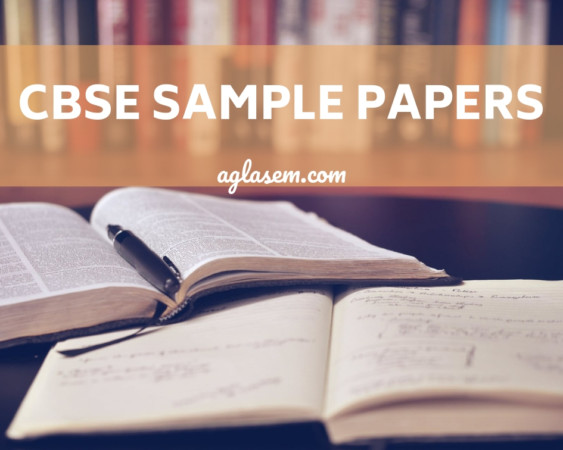CBSE Sample Question Papers for Class 11 Maths Set A with Answers are now available for download in PDF format. CBSE Sample Paper are provided below with answers as per the guidelines of CBSE board marking scheme.

Central Board of Secondary Education Board Exam Sample Question Paper for Class 11 are given here. CBSE Class 11 Maths Sample Paper gives an idea of question paper pattern and marking scheme.

## CBSE Sample Paper for Class 11 Maths (Solved) – Set A

No chapter wise weightage for Class 11 Maths Paper. Care to be taken to cover all the chapters. The above paper is only a sample. Suitable internal variants may be made for generating similar templates keeping the overall weightage to different form of questions and typology of questions same. Set A of Solved CBSE Sample Paper for Class 11 Maths is given below with its solutions.

## Sample Question Paper

Section-A

Question numbers 1 to 6 carry 1 mark each.

1. Let A = {1, 2, 3, 5} and B = {4, 6, 9}. Define a relation R from A to B by R = {(x, y) : x -y is odd natural number, X E A, y E B}. Write R in roster form.

2. Find the domain and range of the function f (x) = 1/√5 – x

3. Evaluate :4. Evaluate the value of cosec (- 1410°).

5. One end of a diameter of a circle is (- 2, – 3) and centre is (2, – 5). Find the coordinates of the other end of the diameter.

6. Write converse of, if two lines are parallel, then they do not intersect in same plane.

Section-B

Question numbers 7 to 19 carry 4 marks each.

7. In any AABC, prove that[Supplementry Material Issued by Board]

OR

Find the value of tan (α + β), given that cot α = 1/2, α∈(π,(3π/2)) and sec β = 5/3, β∈(π/2,π).

8. Prove that : cot(15/2)° = √2 + √3 + √4 + √6.

9. Find the value of k, for which the roots of the equation 2kx – 5k = (k – 3)x2 are real and distinct.

OR

Express in the form of a + ib, ((3 + i√5)(3 – i√5))/((√3) + i√2) – (√3) – i√2))

On her vacations Veena visits four cities (A, B, C and D) in a random order. What is the probability that she visits :

(i) A before B and B before C?

(ii) A first and B last ?

11. Four cards are drawn at random from a pack of 52 playing cards. Find the probability of getting one card from each suit.

12. Using principle of mathematical induction, prove that 4′ + 15n – 1 is divisible by 9, for all n ∈ N.

OR

Using principle of mathematical induction, prove that13. Find the domain and range of the function : f(x) = x2/(x2 + 1) , x ∈ R.

14. Find the derivative of sin (5x – 8) with respect to first principle.

15. Find the domain and range of the function f (x) = 1/(√x – |x|)

16. If a is A.M. and b and c be two G.M.s between any two positive numbers, then prove that b3 + c3 = 2abc.

17. Show that the triangle with vertices (1, 4, 2), (2, – 3, 4) and (- 2, 1, 2) is right angled.

OR

Find the coordinates of the orthocentre of the triangle whose vertices are (-1, 3), (2, – 1) and (0, 0).

18. Find the variance for the following data :

 Classes 0-30 30 – 60 60 – 90 90-120 120-150 150-180 180-210 Frequencies 2 3 5 10 3 5 2

19. How many words, with or without meaning, each of 2 vowels and 3 consonants can be formed from the letters out of word DAUGHTER ?

Section-C

Question numbers 20 to 26 carry 6 marks each.

Prove that : (cos4x+cos3x+cos2x)/(sin4x+sin3x+sin2x) = cot3x

21. In a survey of 5,000 people in a town, 2,250 were listed as reading English newspaper, 1,750 are reading Hindi newspaper and 875 were listed as reading both Hindi as well as English.

Find how many people neither read Hindi nor English newspaper ? Find how many people read only English newspaper ?

22. A family of 4 members planned to go for a Journey to Goa by train during summer for adventures. On the day of Journey all the auto/taxi drivers were on strike due to price hike of petrol. So the family couldn’t get any transport to Railway station. Now family is standing at the crossing of two straight roads represented by equations 2x— 3y — 4 = 0 and 3x + 4y — 5 = 0, want to reach the path whose equation is 6x — 7y + 8 = 0 in least time. Find the equation of path that they should follow and why ? [Value Based Question]

23. If the coefficients of three successive terms in the expansion of (1 + x)n be 45, 120 and 210, find the value of n.

OR

Using binomial theorem, find the value of (52)4.

24. Solve the following system of inequalities :

3x + 3y ≤ 60, x + 3y = ≤ 30, x ≥ 0, y ≥ 0.

25. Show that :26. If (a + ib) (c + id) (e + it) (g + ih) = A + iB , show that (a2 + b2) (c2 + d2) (e2 + f2) (g2 + h2) = A2 + B2.

OR

Describe the set of complex numbers z such that

|(z + 2 – i)/z + 5 + 4i| = 5